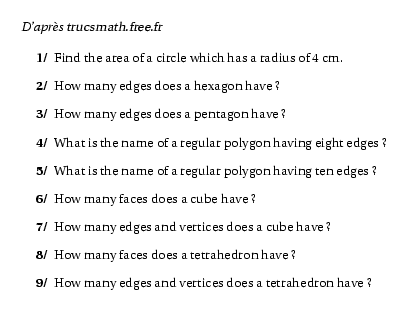Modifié le 19 Octobre 2006 à 21 h 07.Source
%@Auteur: D'après {\sl trucsmath.free.fr}\par
\selectlanguage{english}
\begin{myenumerate}
\item Find the area of a circle which has a radius of 4~cm.
\item How many edges does a hexagon have ?
\item How many edges does a pentagon have ?
\item What is the name of a regular polygon having eight edges ?
\item What is the name of a regular polygon having ten edges ?
\item How many faces does a cube have ?
\item How many edges and vertices does a cube have ?
\item How many faces does a tetrahedron have ?
\item How many edges and vertices does a tetrahedron have ?
\end{myenumerate}
\selectlanguage{frenchb}